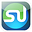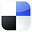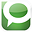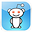# of Average Sales

This article will show you exactly how to calculate a 95% Confidence Interval in Excel of daily sales for a commercial web site. The 95% Confidence Interval is the interval for which we are 95% sure that the true mean of daily sales lies within.

The larger the sample size, the more accurate and smaller the confidence interval will be. We are not taking a random sample in this case, but are using the most recent 60 days of sales data.

The advantages of statistical analysis in Excel to solve business statistics problems is that most problems can be solved in just one or two steps and there is no more need to look anything up on Normal Distribution tables.

The 95% Confidence Interval calculated here is a Confidence Interval for Means, not Proportion. A Confidence Interval for Proportion uses an entirely different set of statistical formulas. Another article in this blog shows exactly how to calculate a Confidence Interval of Proportion. Here is the problem:

Problem: Average daily demand for a product sold on a web site is 455 units sold with a standard deviation of 200. This average and standard deviation are taken from sale data collected every day for the most recent 60 days. What is the range that the true average daily product sales lies in with 95% certainty?

Before we begin solving this problem, we need to know about creating Confidence Intervals in Excel. Here is a brief lesson:

Basic Explanation of Confidence Intervals

The Confidence Interval is an interval in which the true population mean or proportion probably lies based upon a much smaller random sample taken from that population.

A 95% Confidence Interval of a Mean is the interval that has a 95% chance of containing the true population mean.

The width of a Confidence Interval is affected by the sample size. The larger the sample size, the more accurate and tighter is the estimate of the true population mean. The larger the sample size, the smaller will be the Confidence Interval. Samples taken must be random and also be representative of the population.

Calculate Confidence Intervals Using Large Samples (n is greater than 30)

Confidence Intervals are usually calculated and plotted on a Normal curve. If the sample size is less then 30, the population must be known to be Normally distributed. If small-sample data (n  less than 30) is used to plot the Confidence Interval of the Mean for a population that is not Normally distributed, the result can be totally wrong.

Probably the most common major mistake in statistics is to apply Normal or t-distribution tests to small-sample data taken from a population of unknown distribution.

Typically the actual distribution of a population is not known. If the population's underlying distribution is not known (usually it is not), then only large samples (n greater than 30) are valid for creating a Confidence Interval of the Mean. The most important theorem of statistics, the Central Limit Theorem, explains the reason for this.

The Central Limit Theorem

The Central Limit Theorem is statistics' most fundamental theorem. In a nutshell, it states the following: Random sample data can be plotted on a Normal curve to estimate a population's mean no matter how the population is distributed, as long as sample size is large (n greater than 30).

The above definition of the Central Limit Theorem is the most practical and easy-to-understand. The following definition of this theorem is a bit more technical and will satisfy statisticians (but basically says the same thing as the above): No matter how the population is distributed, the sampling distribution of the mean approaches the Normal curve as sample size becomes large.

Confidence Interval of a Population Mean

Creating a Confidence Interval for a population's mean is very similar to creating a Confidence Interval for a population's proportion. The only real difference is how the standard error is calculated. Everything else is the same.

Mean Sampling vs. Proportion Sampling

What determines whether a mean is being estimated or a proportion is being estimated is the number of possible outcomes of each sample taken.

Proportion samples have only two possible outcomes
. For example, if you are comparing the proportion of click-through visitors in two different PPC ads who converted (purchased), each sample has only two possible values; the visitor sampled either converted (purchased) or they didn't.

Mean samples have multiple possible outcomes
. For example, if you are comparing the mean sales of salespeople in two different cities, each salesperson's sales sampled can have numerous values.

Below is a description of how to calculate a Confidence Interval for a population's mean. Note that everything is almost the same as the calculation of the Confidence Interval for a proportion, except sample standard error.

Levels of Confidence and Significance

Level of Significance
, α ("alpha"), equals the maximum allowed percent of error. If the maximum allowed error is 5%, then α = 0.05.

Level of Confidence
is selected by the user. A 95% Level is the most common. A 95% Confidence Level would correspond to a 95% Confidence Interval of the Proportion.

This would state that the actual population Proportion has a 95% probability of lying within the calculated interval. A 95% Confidence Level corresponds to a 5% Level of Significance, or α = 0.05. The Confidence Level therefore equals 1 - α.

Population Proportion vs. Sample Proportion

Population Mean
= µ  (Greek letter "mu")  -  This is what we are trying to estimate.

Sample Mean
= xavg

Sample Size
= n

Standard Deviation and Standard Error

Standard Deviation is a measure of statistical dispersion. It's formula is the following:

SQRT ( [ SUM { (x - xavg)^2 }  ] / n).

There is no need to memorize the formula because you can plug in Excel's STDEV function discussed later. Standard Deviation equals the square root of the Variance.

Population Standard Deviation = σ ("sigma")

Sample Standard Deviation = s

Standard Error
is an estimate of population Standard Deviation from data taken from a sample. If the population Standard Deviation, σ, is known, then the Sample Standard Error, sxavg, can be calculated.

If only the Sample Standard Deviation, s, is known, then Sample Standard Error, sxavg, can be estimated by substituting Sample Standard Deviation, s, for Population Standad Deviation, σ, as follows:

Sample Standard Error = sxavg = σ / SQRT(n) ≈ s / SQRT(n)

Region of Certainty vs. Region of Uncertainty

Region of Certainty
is the area under the Normal curve that corresponds to the required Level of Confidence. If a 95% percent Level of Confidence is required, then the Region of Certainty will contain 95% of the area under the Normal curve. The outer boundaries of the Region of Certainty will be the outer boundaries of the Confidence Interval.

The Region of Certainty, and therefore the Confidence Interval, will be centered about the mean. Half of the Confidence Interval is on one side of the mean and half on the other side.

Region of Uncertainty
is the area under the Normal curve that is outside of the Region of Certainty. Half of the Region of Uncertainty will exist in the right outer tail of the Normal curve and the other half in the left outer tail. This is similar to the concept of the "two-tailed test" that is used in Hypothesis testing in further sections of this course. The concepts of one and two-tailed testing are not used when calculating Confidence Intervals. Just remember that the Region of Certainty, and therefore the Confidence Interval, are always centered about the mean on the Normal curve.

Relationship Between Region of Certainty, Uncertainty, and Alpha

The Region of Uncertainty corresponds to α ("alpha"). If α = 0.05, then that Region of Uncertainty contains 5% of the area under the Normal curve. Half of that area (2.5%) is in each outer tail. The 95% area centered about the mean will be the Region of Certainty. The outer boundaries of this Region of Certainty will be the outer boundaries of the 95% Confidence Interval.

The Level of Confidence is 95% and the Level of Significance, or maximum error allowed, is 5%.

Z Score

Z Score is the number of Standard Errors from the mean to outer right boundary of the Region of Certainty (and therefore to the outer right boundary of the Confidence Interval).

Standard Errors are used and not Standard Deviations because sample data is being used to calculate the Confidence Interval.

Z Score is calculated by the following Excel function:

Z Score(1-α) = NORMSINV (1 - α/2) - This will be discussed shortly.

Excel Functions Used When Calculating Confidence Interval for a Population Mean

Excel functions that are used are listed and are highlighted in BOLD::

COUNT
(Highlighted block of cells) = Sample size = n

This counts the number of cells in highlighted block

NORMSINV
(1 - α/2)  =  Z Score(1 - α)

= Number of Standard Errors from mean to boundary of Confidence Interval.

Note that (1 - α/2)  =  the entire area in the Normal curve to the left of outer right boundary of the Region of Certainty, or Confidence Interval. This includes the entire Region of Certainty and the half of the Region of Uncertainty that exists in the left tail.

For example:

Level of Confidence = 95% for a 95% Confidence Interval

Level of Significance = 5% (α = 0.05)

1 - α = 0.95 = 95%

Z Score95% = NORMSINV (1 - α/2) = NORMSINV (1 - .05/2) = NORMSINV(1 - 0.025)

Z Score95% = NORMSINV (0.975) = 1.96

The outer right boundary of the 95% Confidence Interval, and the Region of Certainty, is 1.96 Standard Errors from the mean. The left boundary is the same distance from the mean because the Confidence Interval is centered about the mean.

Formulas for Calculating Confidence Interval Boundaries from Sample Data for a Population Mean

Confidence Interval Boundaries
= Sample proportion +/- Z Score(1-α) * Sample Standard Error

Confidence Interval Boundaries = xavg +/- Z Score(1-α) * sxavg

Sample Mean = xavg

Z Score(1 - α) = NORMSINV (1 - α/2)

Sample size = n = COUNT (Highlighted block of cells containing samples)

Confidence Interval Boundaries = xavg +/- Z Score(1-α) * sxavg

COUNT
(Highlighted block of cells) = Sample size = n

- Counts number of cells in highlighted block

STDEV (Highlighted block of cells) = Standard deviation
- Calculates Standard Deviation of all cells in highlighted block

AVERAGE (Highlighted block of cells) = Mean
- Calculates the mean of all cells in highlighted block

NORMSINV (1 - α/2) = Z Score(1 - α)

= Number of Standard errors from mean to boundary of Confidence Interval. Note that (1 - α/2)

= the entire area in the Normal curve to the left of outer right boundary of the Region of Certainty, or Confidence Interval. This includes the entire Region of Certainty and the half of the Region of Uncertainty that exists in the left tail.

For example:

Level of Confidence = 95% for a 95% Confidence Interval

Level of Significance = 5% (α = 0.05)

1 - α = 0.95 = 95%

Z Score95% = NORMSINV(1 – α/2) = NORMSINV (1 - .05/2) = NORMSINV(1 - 0.025)

Z Score95% = NORMSINV (0.975) = 1.96

The outer right boundary of the 95% Confidence Interval, and the Region of Certainty, is 1.96 Standard Errors from the mean. The left boundary is the same distance from the mean because the Confidence Interval is centered about the mean.

CONFIDENCE ( α, s, n ) = Width of half of the Confidence Interval

α = Level of Significance
s = Sample Standard Deviation - Note that this is not Standard Error.
s is calculated by applying STDEV to the sample values.
n = Sample size - Apply COUNT to sample values.

Confidence Interval Boundaries = Sample mean +/- Z Score(1-α) * Sample Standard Error

Confidence Interval Boundaries = xavg +/- Z Score(1-α) * sxavg

Sample Mean
= xavg = AVERAGE (Highlighted block of cell containing samples)

Z Score(1 - α) = NORMSINV (1 - α/2)

Sample Standard Error = sxavg = σ / SQRT(n)  ≈ s / SQRT(n)

Sample Size = n = COUNT (Highlighted block of cells containing samples)

Sample Standard Deviation = s = STDEV (Highlighted block of cells containing samples)

CONFIDENCE ( α, s, n ) = Width of half of the Confidence Interval

CONFIDENCE
( α, s, n ) = Z Score(1-α) * sxavg

So:

Confidence Interval Boundaries
= xavg +/- Z Score(1-α) * sxavg

Confidence Interval Boundaries

= xavg +/- CONFIDENCE ( α, s, n )

Click On Image To See Larger Version

*******************************************************************
Now we're ready to create a Confidence Interval of Mean to solve the following problem:

Problem: Calculate a Confidence Interval of Daily Internet Sales from a Single Web Site Based Upon Mean and Standard Deviation from a Sample of the most recent Daily Sales.

Average daily demand for a product sold on a web site is 455 units sold with a standard deviation of 200. This average and standard deviation are taken from sale data collected every day for the most recent 60 days. What is the range that the true average daily product sales lies in with 95% certainty?

Level of Significance = α = 0.05

Sample Size = n = 60

Sample Mean = xavg = 455

Sample Standard Deviation = s = 200

Sample Standard Error = sxavg = σ / SQRT(n) ≈ s / SQRT(n)

sxavgs / SQRT(n) = 200 / SQRT(60) = 25.8

Z Score(1 - α) = Z Score95% = NORMSINV (1 - α/2)

= NORMSINV (1 - 0.025) = NORMSINV (0.975) = 1.96

Width of Half the Confidence Interval = CONFIDENCE ( α, s, n)

= CONFIDENCE ( 0.05, 200, 60) = 50.6

Width of Half the Confidence Interval = Z Score(1-α) * sxavg

= 1.96 * 25.8 = 50.6

Confidence Interval Boundaries = xavg +/- Z Score(1-α) * sxavg

= 455 +/- (1.96)*(25.8) = 455 +/- 50.6 = 404.4 to 505.6

Also, equivalently:

Confidence Interval Boundaries = xavg +/- CONFIDENCE ( α, s, n)

= 455 +/- 50.6 = 404.4 to 505.6

Click Image To See Larger Version

*****************************************

Create a Confidence Interval in Excel To Find Your True Daily Sales

If You Like This, Then Share It...Excel Master Series Blog Directory

Statistical Topics and Articles In Each Topic

• Histograms in Excel
• Bar Chart in Excel
• Combinations & Permutations in Excel
• Normal Distribution in Excel
• t-Distribution in Excel
• Binomial Distribution in Excel
• z-Tests in Excel
• t-Tests in Excel
• Hypothesis Tests of Proportion in Excel
• Chi-Square Independence Tests in Excel
• Chi-Square Goodness-Of-Fit Tests in Excel
• F Tests in Excel
• Correlation in Excel
• Pearson Correlation in Excel
• Spearman Correlation in Excel
• Confidence Intervals in Excel
• Simple Linear Regression in Excel
• Multiple Linear Regression in Excel
• Logistic Regression in Excel
• Single-Factor ANOVA in Excel
• Two-Factor ANOVA With Replication in Excel
• Two-Factor ANOVA Without Replication in Excel
• Creating Interactive Graphs of Statistical Distributions in Excel
• Solving Problems With Other Distributions in Excel
• Optimization With Excel Solver
• Chi-Square Population Variance Test in Excel
• Analyzing Data With Pivot Tables
• SEO Functions in Excel
• Time Series Analysis in Excel

#### 1 comment:

1.What is the best Sega Genesis game? - Casino Sites
This list of the top-rated video game consoles 바카라사이트 and the games that best fit into casinosites one